# NCERT Exemplar Class 11 Chemistry Solutions for Chapter 4 - Chemical Bonding and Molecular Structure

## NCERT Exemplar Solutions Class 11 Chemistry Chapter 4 – Free PDF Download

NCERT Exemplar Solutions for Class 11 Chemistry Chapter 4 Chemical Bonding and Molecular Structure are provided here to help students develop a clear understanding of chemical bonding. Chemical Bonding and Molecular Structure is a very important topic that lays the foundation for your future studies. In order to understand the topic clearly, students are advised to practise the exemplar solutions provided here. This page has NCERT Exemplar Solutions for Class 11 Chemistry Chapter 4 Chemical Bonding, along with important questions from previous years’ question papers, worksheets, exercises, tips and tricks that will help you score high in CBSE Class 11 as well as entrance examinations. The exemplar solutions have been prepared by subject experts who have included detailed answers in simple language and style to help students remember and understand each topic easily.

Chapter 4, which deals with the topic of chemical bonds and molecular structure, is included in the Class 11 Chemistry textbook to help students develop a clear understanding of chemical bonding. In this chapter, they have to examine the formation of different types of bonds, like a covalent bond or hydrogen bond, understand different rules and theories, and hybridisation. To help students develop a strong foundation and master the chapter easily, NCERT Exemplar Solutions for Class 11 Chemistry Chapter 4 – Chemical Bonding and Molecular Structure are available here for free download. Students can use this exemplar as a reference tool to study effectively and to score high grades in the annual exam. The NCERT Exemplar is available in PDF in the link below, and students can download and use it at their convenience.

## Download the PDF of NCERT Exemplar for Class 11 Chemistry Chapter 4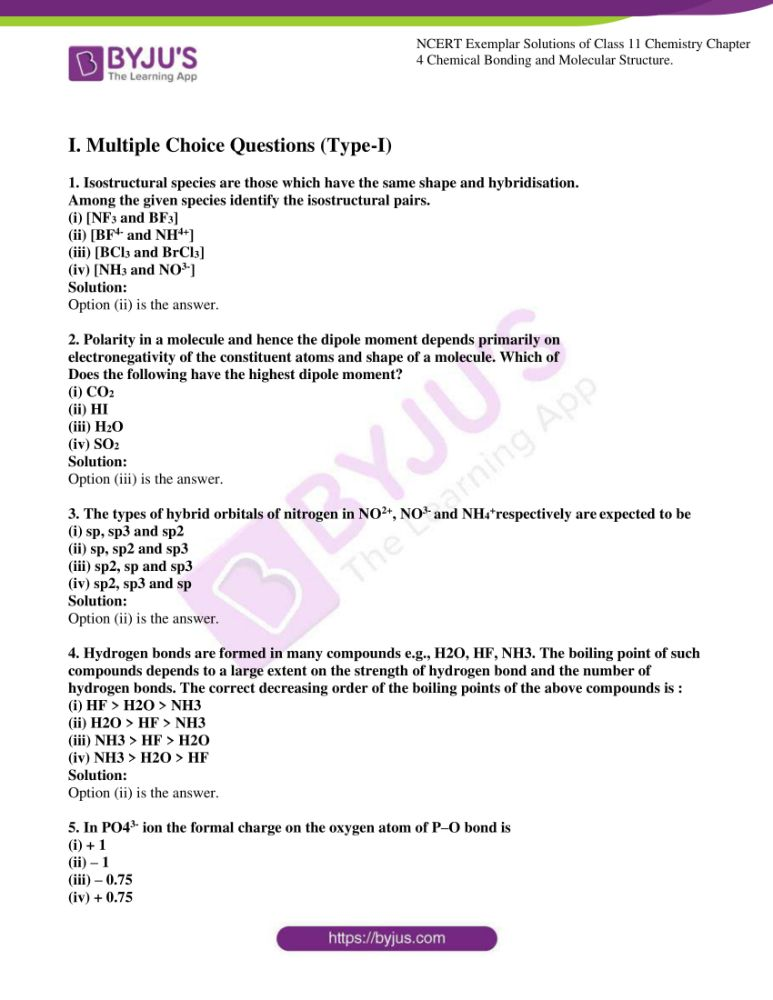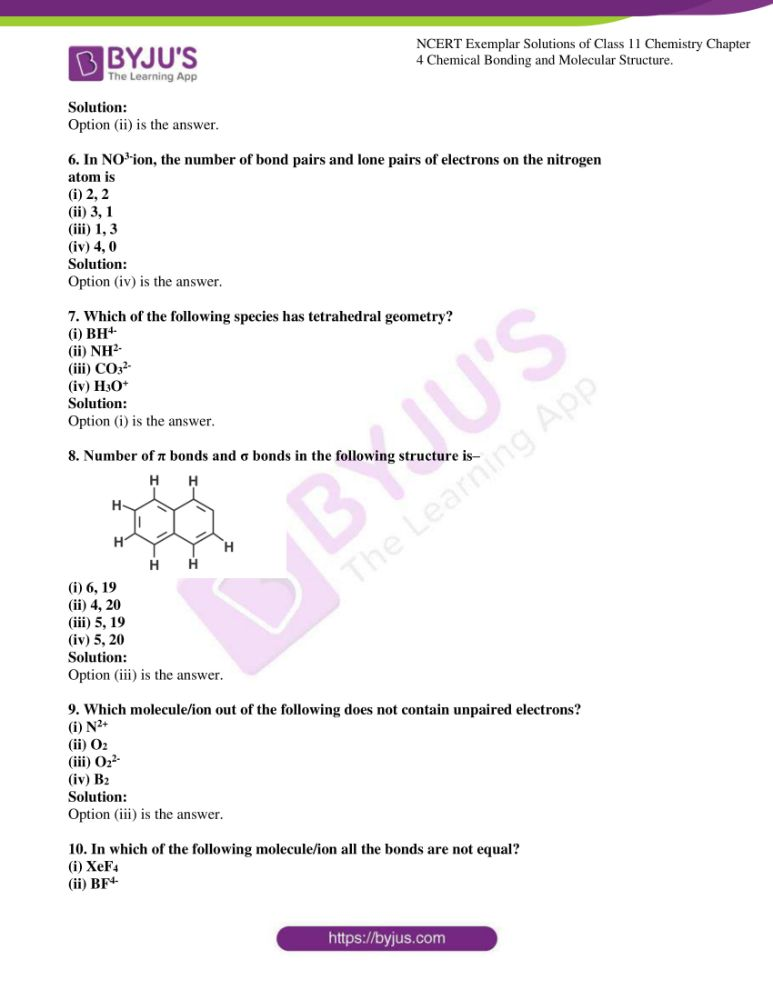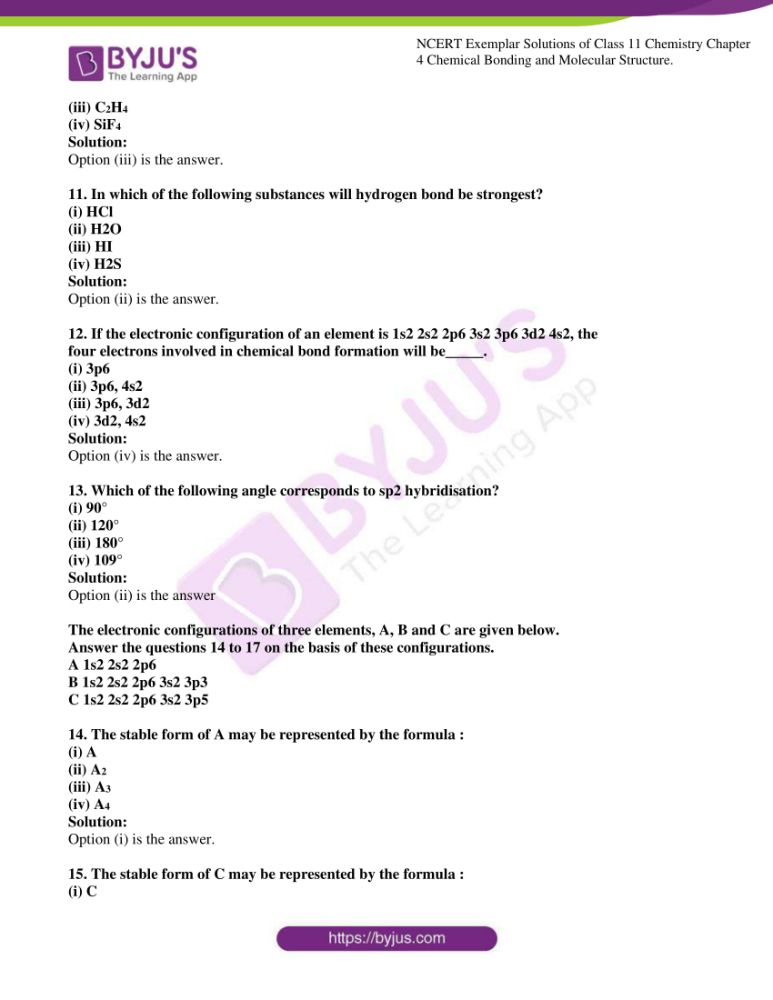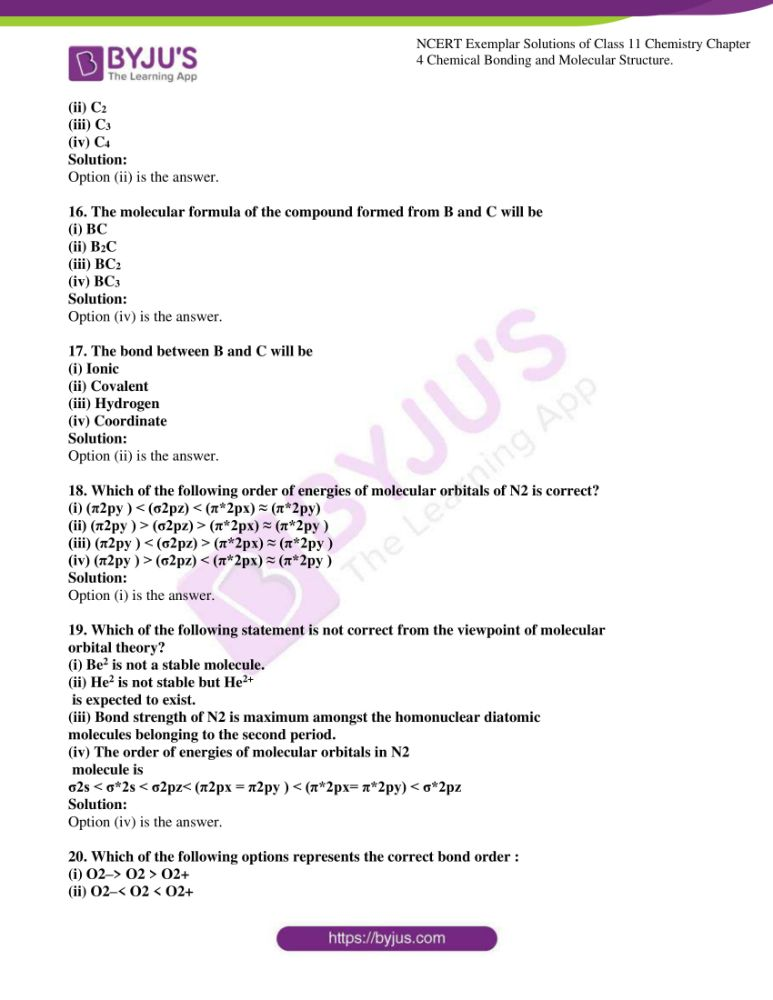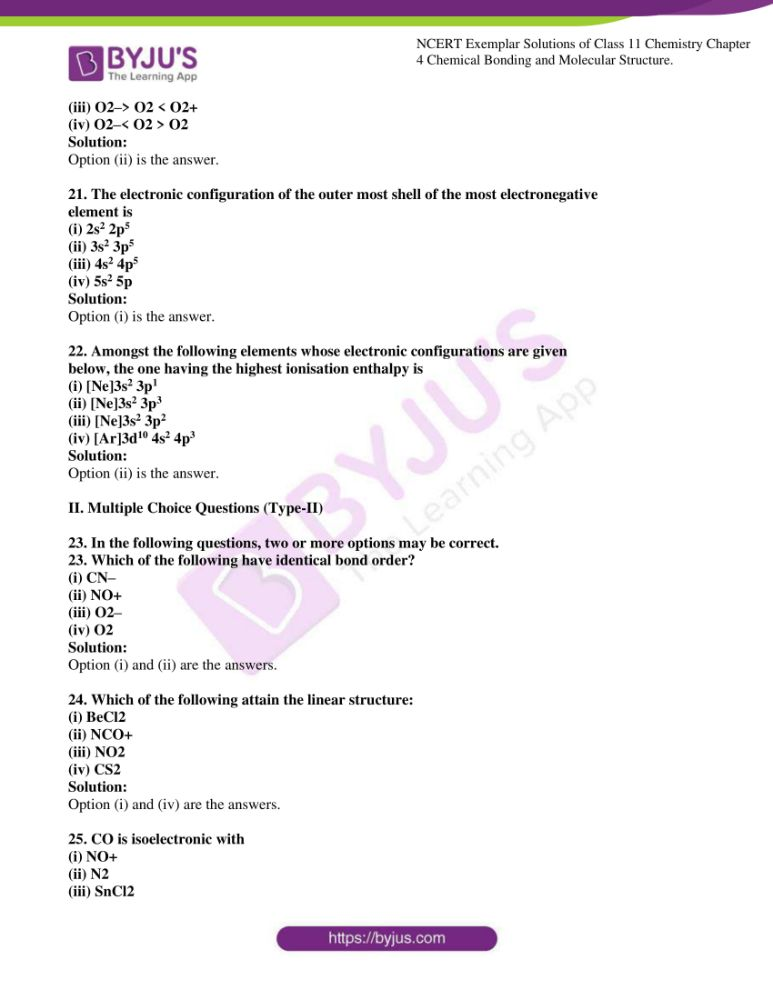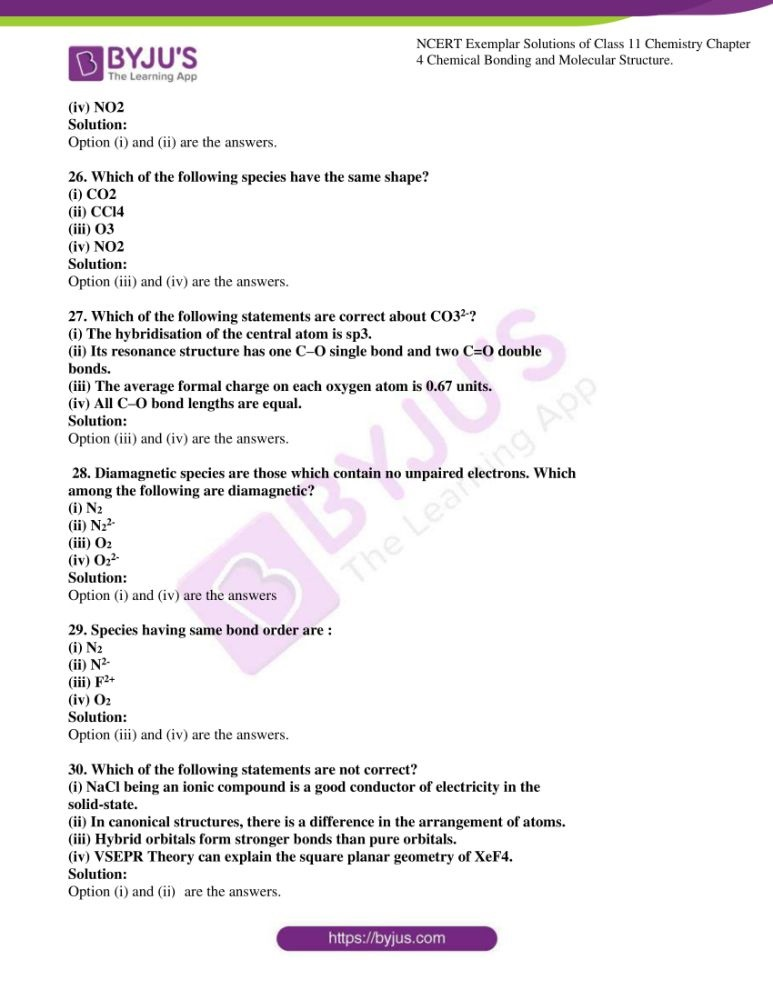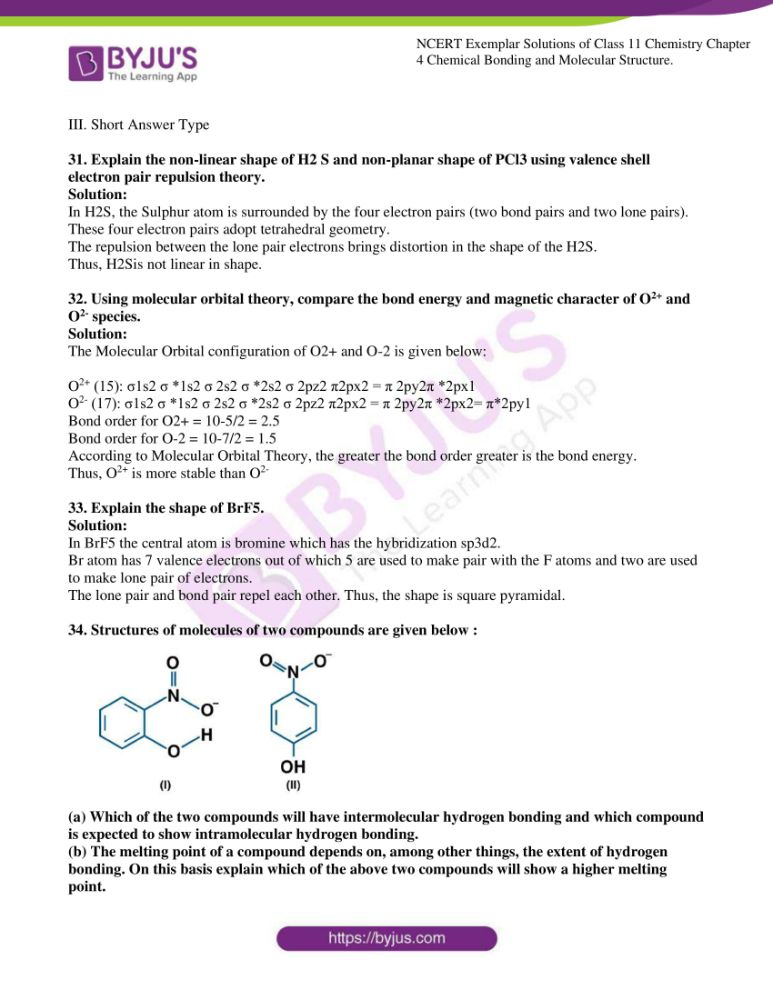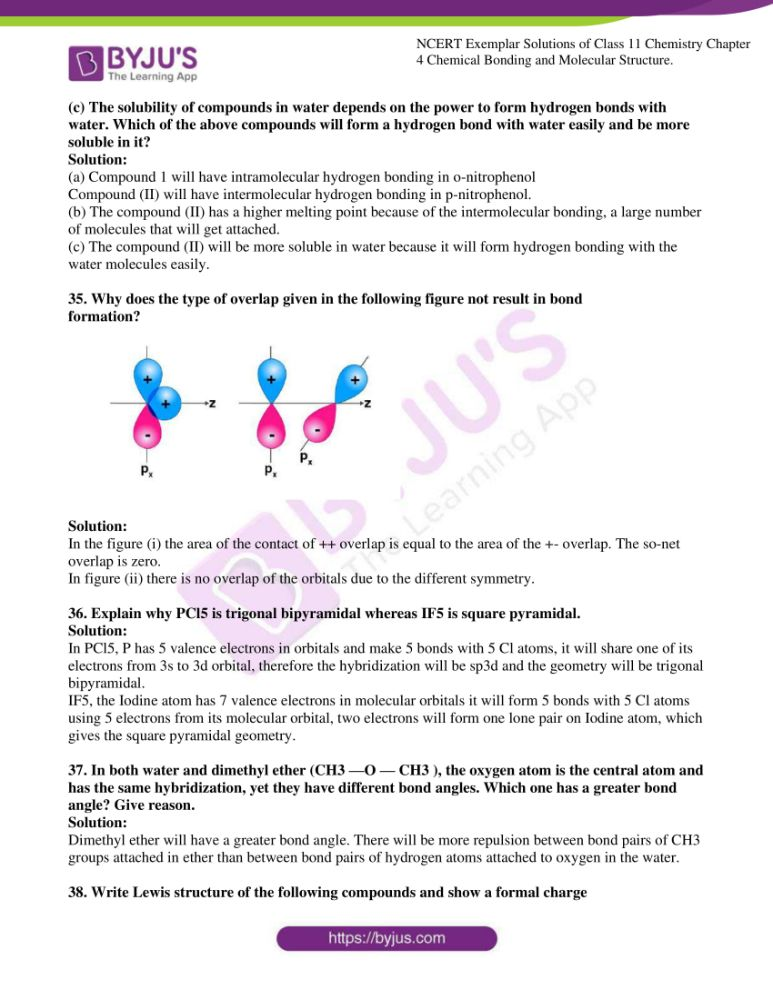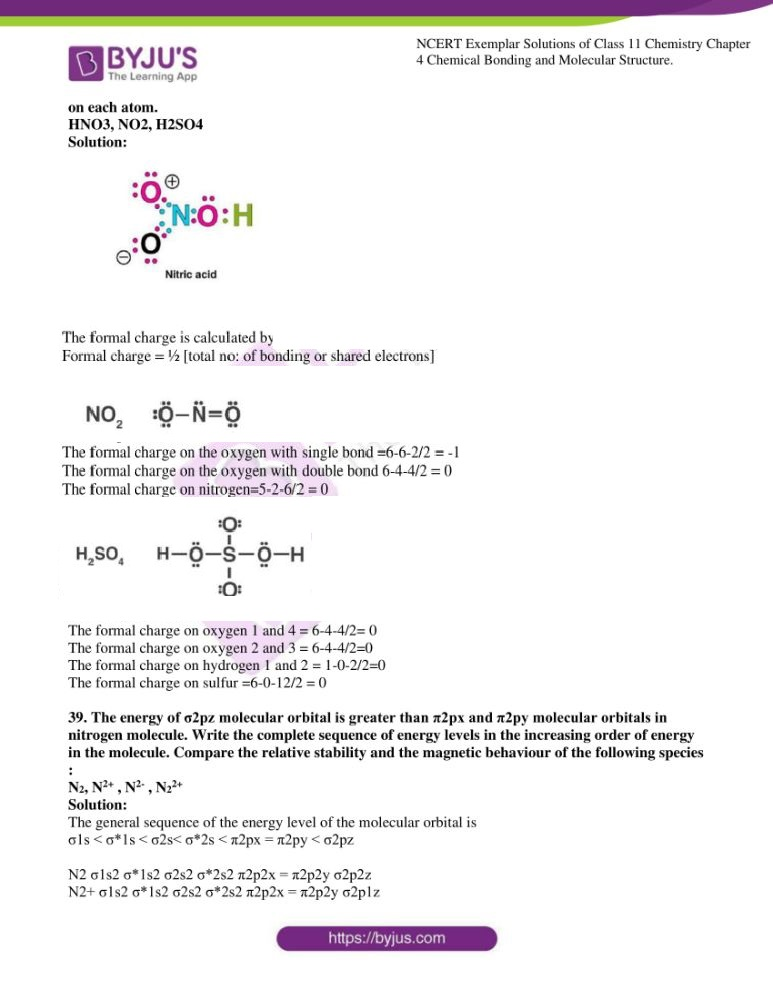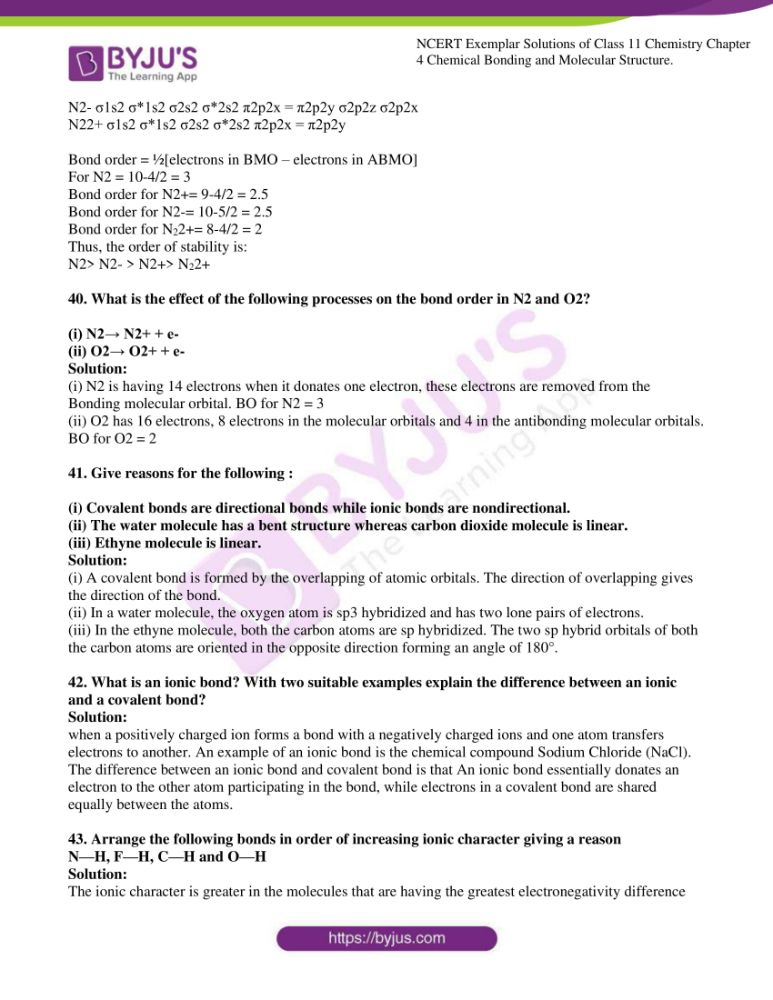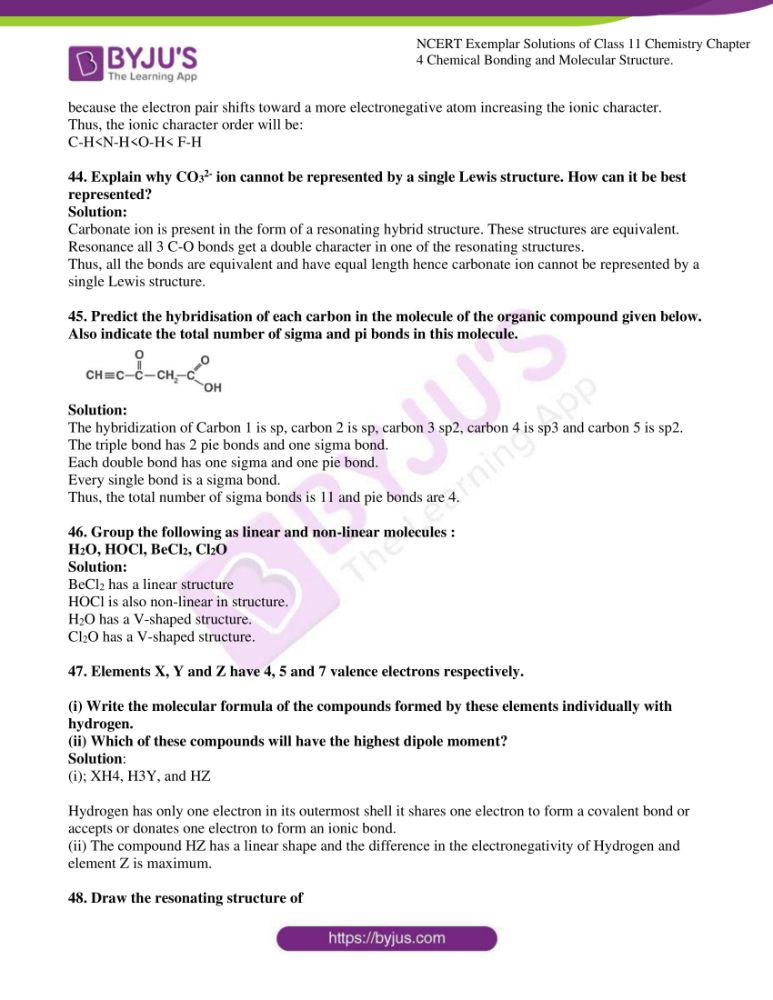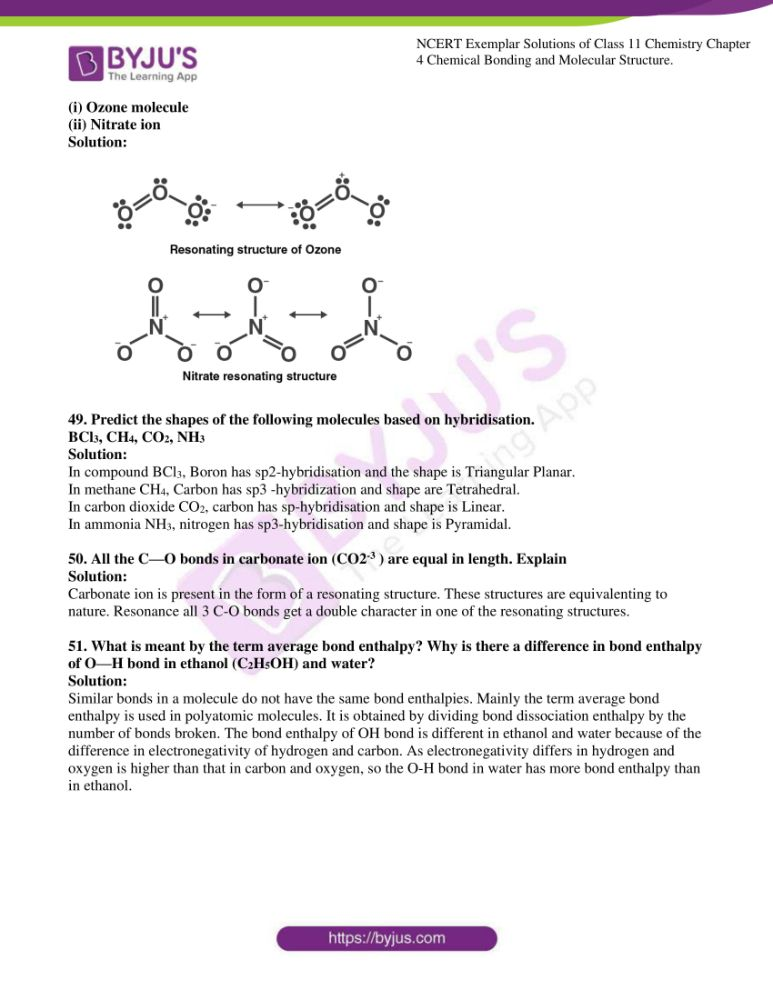### Access Answers to NCERT Exemplar Solutions for Class 11 Chemistry Chapter 4

I. Multiple-choice Questions (Type-I)

1. Isostructural species are those which have the same shape and hybridisation.

Among the given species, identify the isostructural pairs.

(i) [NF3 and BF3]

(ii) [BF4- and NH4+]

(iii) [BCl3 and BrCl3]

(iv) [NH3 and NO3-]

Solution:

2. Polarity in a molecule and hence the dipole moment depends primarily on

electronegativity of the constituent atoms and shape of a molecule. Which of

Does the following have the highest dipole moment?

(i) CO2

(ii) HI

(iii) H2O

(iv) SO2

Solution:

3. The types of hybrid orbitals of nitrogen in NO2+, NO3- and NH4+respectively are expected to be

(i) sp, sp3 and sp2

(ii) sp, sp2 and sp3

(iii) sp2, sp and sp3

(iv) sp2, sp3 and sp

Solution:

4. Hydrogen bonds are formed in many compounds e.g., H2O, HF, NH3. The boiling point of such compounds depends to a large extent on the strength of hydrogen bond and the number of hydrogen bonds. The correct decreasing order of the boiling points of the above compounds is :

(i) HF > H2O > NH3

(ii) H2O > HF > NH3

(iii) NH3 > HF > H2O

(iv) NH3 > H2O > HF

Solution:

5. In PO43- ion, the formal charge on the oxygen atom of P–O bond is

(i) + 1

(ii) – 1

(iii) – 0.75

(iv) + 0.75

Solution:

6. In NO3-ion, the number of bond pairs and lone pairs of electrons on the nitrogen

atom is

(i) 2, 2

(ii) 3, 1

(iii) 1, 3

(iv) 4, 0

Solution:

7. Which of the following species has tetrahedral geometry?

(i) BH4-

(ii) NH2-

(iii) CO32-

(iv) H3O+

Solution:

8. Number of π bonds and σ bonds in the following structure is–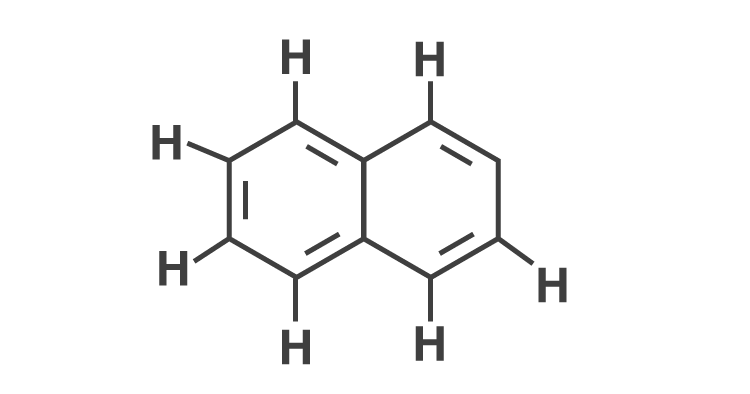(i) 6, 19

(ii) 4, 20

(iii) 5, 19

(iv) 5, 20

Solution:

9. Which molecule/ion out of the following does not contain unpaired electrons?

(i) N2+

(ii) O2

(iii) O22-

(iv) B2

Solution:

10. In which of the following molecules/ions all the bonds are not equal?

(i) XeF4

(ii) BF4-

(iii) C2H4

(iv) SiF4

Solution:

11. In which of the following substances will the hydrogen bond be strongest?

(i) HCl

(ii) H2O

(iii) HI

(iv) H2S

Solution:

12. If the electronic configuration of an element is 1s2 2s2 2p6 3s2 3p6 3d2 4s2, the

four electrons involved in chemical bond formation will be_____.

(i) 3p6

(ii) 3p6, 4s2

(iii) 3p6, 3d2

(iv) 3d2, 4s2

Solution:

13. Which of the following angle corresponds to sp2 hybridisation?

(i) 90°

(ii) 120°

(iii) 180°

(iv) 109°

Solution:

The electronic configurations of three elements, A, B and C are given below.

Answer questions 14 to 17 on the basis of these configurations.

A 1s2 2s2 2p6

B 1s2 2s2 2p6 3s2 3p3

C 1s2 2s2 2p6 3s2 3p5

14. The stable form of A may be represented by the formula :

(i) A

(ii) A2

(iii) A3

(iv) A4

Solution:

15. The stable form of C may be represented by the formula :

(i) C

(ii) C2

(iii) C3

(iv) C4

Solution:

16. The molecular formula of the compound formed from B and C will be

(i) BC

(ii) B2C

(iii) BC2

(iv) BC3

Solution:

17. The bond between B and C will be

(i) Ionic

(ii) Covalent

(iii) Hydrogen

(iv) Coordinate

Solution:

18. Which of the following order of energies of molecular orbitals of N2 is correct?

(i) (π2py ) < (σ2pz) < (π*2px) ≈ (π*2py)

(ii) (π2py ) > (σ2pz) > (π*2px) ≈ (π*2py )

(iii) (π2py ) < (σ2pz) > (π*2px) ≈ (π*2py )

(iv) (π2py ) > (σ2pz) < (π*2px) ≈ (π*2py )

Solution:

19. Which of the following statement is not correct from the viewpoint of molecular

orbital theory?

(i) Be2 is not a stable molecule.

(ii) He2 is not stable but He2+

is expected to exist.

(iii) Bond strength of N2 is maximum amongst the homonuclear diatomic

molecules belonging to the second period.

(iv) The order of energies of molecular orbitals in N2

molecule is

σ2s < σ*2s < σ2pz< (π2px = π2py ) < (π*2px= π*2py) < σ*2pz

Solution:

20. Which of the following options represents the correct bond order :

(i) O2–> O2 > O2+

(ii) O2–< O2 < O2+

(iii) O2–> O2 < O2+

(iv) O2–< O2 > O2

Solution:

21. The electronic configuration of the outermost shell of the most electronegative

element is

(i) 2s2 2p5

(ii) 3s2 3p5

(iii) 4s2 4p5

(iv) 5s2 5p

Solution:

22. Amongst the following elements whose electronic configurations are given

below, the one having the highest ionisation enthalpy is

(i) [Ne]3s2 3p1

(ii) [Ne]3s2 3p3

(iii) [Ne]3s2 3p2

(iv) [Ar]3d10 4s2 4p3

Solution:

II. Multiple Choice Questions (Type-II)

In the following questions, two or more options may be correct.

23. Which of the following has identical bond order?

(i) CN–

(ii) NO+

(iii) O2–

(iv) O2

Solution:

Option (i) and (ii) are the answers.

24. Which of the following attains the linear structure:

(i) BeCl2

(ii) NCO+

(iii) NO2

(iv) CS2

Solution:

Option (i) and (iv) are the answers.

25. CO is isoelectronic with

(i) NO+

(ii) N2

(iii) SnCl2

(iv) NO2

Solution:

Option (i) and (ii) are the answers.

26. Which of the following species have the same shape?

(i) CO2

(ii) CCl4

(iii) O3

(iv) NO2

Solution:

Option (iii) and (iv) are the answers.

27. Which of the following statements are correct about CO32-?

(i) The hybridisation of the central atom is sp3.

(ii) Its resonance structure has one C–O single bond and two C=O double

bonds.

(iii) The average formal charge on each oxygen atom is 0.67 units.

(iv) All C–O bond lengths are equal.

Solution:

Option (iii) and (iv) are the answers.

28. Diamagnetic species are those which contain no unpaired electrons. Which

among the following are diamagnetic?

(i) N2

(ii) N22-

(iii) O2

(iv) O22-

Solution:

Option (i) and (iv) are the answers

29. Species having the same bond order are :

(i) N2

(ii) N2-

(iii) F2+

(iv) O2

Solution:

Option (iii) and (iv) are the answers.

30. Which of the following statements are not correct?

(i) NaCl being an ionic compound is a good conductor of electricity in the

solid-state.

(ii) In canonical structures, there is a difference in the arrangement of atoms.

(iii) Hybrid orbitals form stronger bonds than pure orbitals.

(iv) VSEPR Theory can explain the square planar geometry of XeF4.

Solution:

Option (i) and (ii) are the answers.

31. Explain the non-linear shape of H2 S and the non-planar shape of PCl3 using valence shell electron pair repulsion theory.

Solution:

In H2S, the Sulphur atom is surrounded by four electron pairs (two bond pairs and two lone pairs).

These four electron pairs adopt tetrahedral geometry.

The repulsion between the lone pair electrons brings distortion in the shape of the H2S.

Thus, H2S is not linear in shape.

32. Using molecular orbital theory, compare the bond energy and magnetic character of O2+ and O2- species.

Solution:

The Molecular Orbital configuration of O2+ and O-2 is given below:

O2+ (15): σ1s2 σ *1s2 σ 2s2 σ *2s2 σ 2pz2 π2px2 = π 2py2π *2px1

O2- (17): σ1s2 σ *1s2 σ 2s2 σ *2s2 σ 2pz2 π2px2 = π 2py2π *2px2= π*2py1

Bond order for O2+ = 10-5/2 = 2.5

Bond order for O-2 = 10-7/2 = 1.5

According to Molecular Orbital Theory, the greater the bond order greater is the bond energy.

Thus, O2+ is more stable than O2-

33. Explain the shape of BrF5.

Solution:

In BrF5, the central atom is bromine which has the hybridization sp3d2.

Br atom has 7 valence electrons, out of which 5 are used to make pair with the F atoms, and two are used to make lone pair of electrons.

The lone pair and bond pair repel each other. Thus, the shape is square pyramidal.

34. Structures of molecules of two compounds are given below :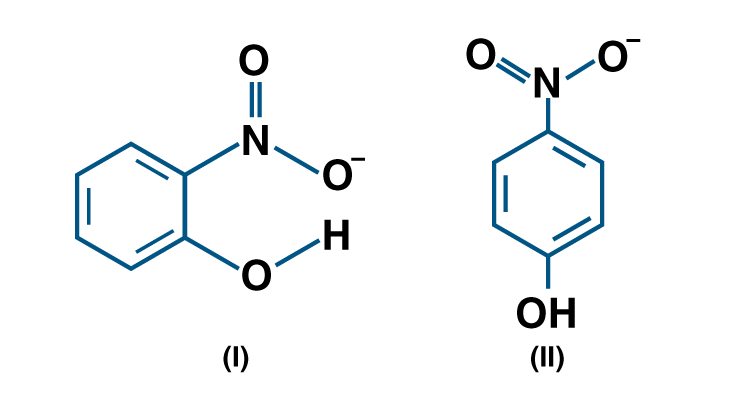(a) Which of the two compounds will have intermolecular hydrogen bonding and which compound is expected to show intramolecular hydrogen bonding.

(b) The melting point of a compound depends on, among other things, the extent of hydrogen bonding. On this basis explain which of the above two compounds will show a higher melting point.

(c) The solubility of compounds in water depends on the power to form hydrogen bonds with water. Which of the above compounds will form a hydrogen bond with water easily and be more soluble in it?

Solution:

(a) Compound 1 will have intramolecular hydrogen bonding in o-nitrophenol

Compound (II) will have intermolecular hydrogen bonding in p-nitrophenol.

(b) The compound (II) has a higher melting point because of the intermolecular bonding, a large number of molecules that will get attached.

(c) The compound (II) will be more soluble in water because it will form hydrogen bonding with the water molecules easily.

35. Why does the type of overlap given in the following figure not result in bond

formation?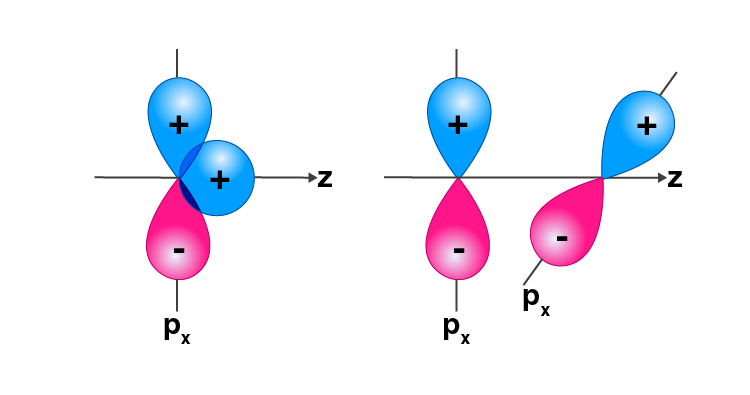Solution:

In the figure (i) the area of the contact of ++ overlap is equal to the area of the +- overlap. The so-net overlap is zero.

In figure (ii) there is no overlap of the orbitals due to the different symmetry.

36. Explain why PCl5 is trigonal bipyramidal whereas IF5 is square pyramidal.

Solution:

In PCl5, P has 5 valence electrons in orbitals and makes 5 bonds with 5 Cl atoms, it will share one of its electrons from 3s to 3d orbital, therefore the hybridization will be sp3d and the geometry will be trigonal bipyramidal.

IF5, the Iodine atom has 7 valence electrons in molecular orbitals it will form 5 bonds with 5 Cl atoms using 5 electrons from its molecular orbital, two electrons will form one lone pair on Iodine atom, which gives the square pyramidal geometry.

37. In both water and dimethyl ether (CH3 —Ο — CH3 ), the oxygen atom is the central atom and has the same hybridization, yet they have different bond angles. Which one has a greater bond angle? Give reason.

Solution:

Dimethyl ether will have a greater bond angle. There will be more repulsion between bond pairs of CH3 groups attached in ether than between bond pairs of hydrogen atoms attached to oxygen in the water.

38. Write Lewis structure of the following compounds and show a formal charge

on each atom.

HNO3, NO2, H2SO4

Solution: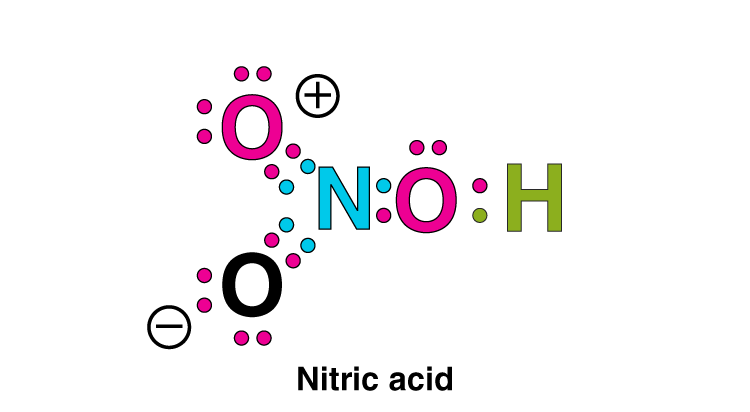The formal charge is calculated by

Formal charge = ½ [total no: of bonding or shared electrons]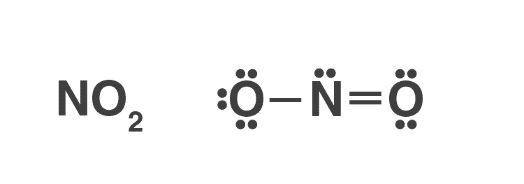The formal charge on the oxygen with single bond =6-6-2/2 = -1

The formal charge on the oxygen with double bond 6-4-4/2 = 0

The formal charge on nitrogen=5-2-6/2 = 0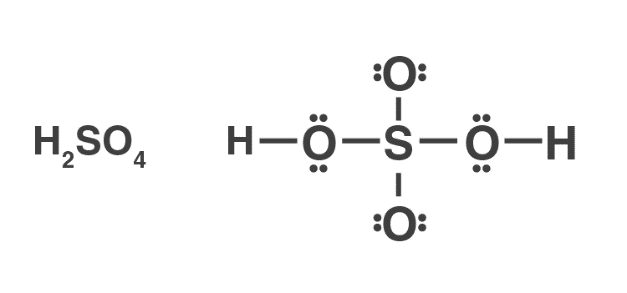The formal charge on oxygen 1 and 4 = 6-4-4/2= 0

The formal charge on oxygen 2 and 3 = 6-4-4/2=0

The formal charge on hydrogen 1 and 2 = 1-0-2/2=0

The formal charge on sulfur =6-0-12/2 = 0

39. The energy of σ2pz molecular orbital is greater than π2px and π2py molecular orbitals in nitrogen molecule. Write the complete sequence of energy levels in the increasing order of energy in the molecule. Compare the relative stability and the magnetic behaviour of the following species :

N2, N2+ , N2- , N22+

Solution:

The general sequence of the energy level of the molecular orbital is

σ1s < σ*1s < σ2s< σ*2s < π2px = π2py < σ2pz

N2 σ1s2 σ*1s2 σ2s2 σ*2s2 π2p2x = π2p2y σ2p2z

N2+ σ1s2 σ*1s2 σ2s2 σ*2s2 π2p2x = π2p2y σ2p1z

N2- σ1s2 σ*1s2 σ2s2 σ*2s2 π2p2x = π2p2y σ2p2z σ2p2x

N22+ σ1s2 σ*1s2 σ2s2 σ*2s2 π2p2x = π2p2y

Bond order = ½[electrons in BMO – electrons in ABMO]

For N2 = 10-4/2 = 3

Bond order for N2+= 9-4/2 = 2.5

Bond order for N2-= 10-5/2 = 2.5

Bond order for N22+= 8-4/2 = 2

Thus, the order of stability is:

N2> N2- > N2+> N22+

40. What is the effect of the following processes on the bond order in N2 and O2?

(i) N2→ N2+ + e-

(ii) O2→ O2+ + e-

Solution:

(i) N2 is having 14 electrons when it donates one electron, these electrons are removed from the Bonding molecular orbital. BO for N2 = 3

(ii) O2 has 16 electrons, 8 electrons in the molecular orbitals and 4 in the antibonding molecular orbitals.

BO for O2 = 2

41. Give reasons for the following :

(i) Covalent bonds are directional bonds while ionic bonds are nondirectional.

(ii) The water molecule has a bent structure whereas carbon dioxide molecule is linear.

(iii) Ethyne molecule is linear.

Solution:

(i) A covalent bond is formed by the overlapping of atomic orbitals. The direction of overlapping gives the direction of the bond.

(ii) In a water molecule, the oxygen atom is sp3 hybridized and has two lone pairs of electrons.

(iii) In the ethyne molecule, both the carbon atoms are sp hybridized. The two sp hybrid orbitals of both the carbon atoms are oriented in the opposite direction forming an angle of 180°.

42. What is an ionic bond? With two suitable examples, explain the difference between an ionic and a covalent bond.

Solution:

when a positively charged ion forms a bond with a negatively charged ions and one atom transfers electrons to another. An example of an ionic bond is the chemical compound Sodium Chloride (NaCl).

The difference between an ionic bond and covalent bond is that An ionic bond essentially donates an electron to the other atom participating in the bond, while electrons in a covalent bond are shared equally between the atoms.

43. Arrange the following bonds in order of increasing ionic character giving a reason

N—H, F—H, C—H and O—H

Solution:

The ionic character is greater in the molecules that have the greatest electronegativity difference because the electron pair shifts toward a more electronegative atom, increasing the ionic character.

Thus, the ionic character order will be:

C-H<N-H<O-H< F-H

44. Explain why CO32- ion cannot be represented by a single Lewis structure. How can it be best represented?

Solution:

Carbonate ion is present in the form of a resonating hybrid structure. These structures are equivalent. Resonance all 3 C-O bonds get a double character in one of the resonating structures.

Thus, all the bonds are equivalent and have equal length hence carbonate ion cannot be represented by a single Lewis structure.

45. Predict the hybridisation of each carbon in the molecule of the organic compound given below. Also indicate the total number of sigma and pi bonds in this molecule.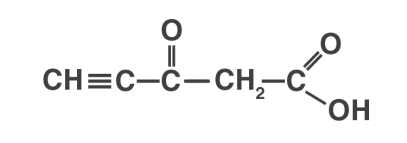Solution:

The hybridization of Carbon 1 is sp, carbon 2 is sp, carbon 3 sp2, carbon 4 is sp3 and carbon 5 is sp2.

The triple bond has 2 pie bonds and one sigma bond.

Each double bond has one sigma and one pie bond.

Every single bond is a sigma bond.

Thus, the total number of sigma bonds is 11 and pie bonds are 4.

46. Group the following as linear and non-linear molecules :

H2O, HOCl, BeCl2, Cl2O

Solution:

BeCl2 has a linear structure

HOCl is also non-linear in structure.

H2O has a V-shaped structure.

Cl2O has a V-shaped structure.

47. Elements X, Y and Z have 4, 5 and 7 valence electrons respectively.

(i) Write the molecular formula of the compounds formed by these elements individually with hydrogen.

(ii) Which of these compounds will have the highest dipole moment?

Solution:

(i); XH4, H3Y, and HZ

Hydrogen has only one electron in its outermost shell it shares one electron to form a covalent bond or accepts or donates one electron to form an ionic bond.

(ii) The compound HZ has a linear shape and the difference in the electronegativity of Hydrogen and element Z is maximum.

48. Draw the resonating structure of

(i) Ozone molecule

(ii) Nitrate ion

Solution: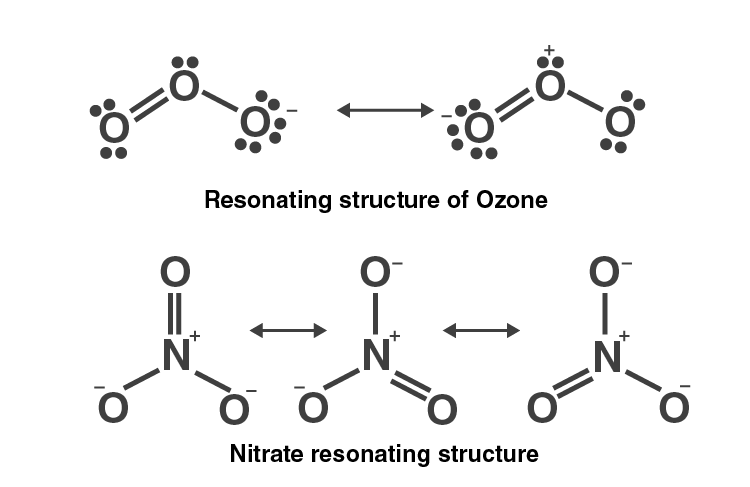49. Predict the shapes of the following molecules based on hybridisation.

BCl3, CH4, CO2, NH3

Solution:

In compound BCl3, Boron has sp2-hybridisation and the shape is Triangular Planar.

In methane CH4, Carbon has sp3 -hybridization and shape are Tetrahedral.

In carbon dioxide CO2, carbon has sp-hybridisation and shape is Linear.

In ammonia NH3, nitrogen has sp3-hybridisation and shape is Pyramidal.

50. All the C—O bonds in carbonate ion (CO2-3 ) are equal in length. Explain

Solution:

Carbonate ion is present in the form of a resonating structure. These structures are equivalenting to nature. Resonance all 3 C-O bonds get a double character in one of the resonating structures.

51. What is meant by the term average bond enthalpy? Why is there a difference in bond enthalpy of O—H bond in ethanol (C2H5OH) and water?

Solution:

Similar bonds in a molecule do not have the same bond enthalpies. Mainly the term average bond enthalpy is used in polyatomic molecules. It is obtained by dividing bond dissociation enthalpy by the number of bonds broken. The bond enthalpy of the OH bond is different in ethanol and water because of the difference in the electronegativity of hydrogen and carbon. As electronegativity differs in hydrogen and oxygen is higher than that in carbon and oxygen, so the O-H bond in water has more bond enthalpy than in ethanol.

#### Important Topics of Class 11 Chemistry Chapter 4 Chemical Bonding and Molecular Structure

1. Octet Rule
1. Covalent Bond
2. Lewis Representation of Simple Molecules (The Lewis Structures)
3. Formal Charge
4. Limitations of the Octet Rule
2. Ionic or Electrovalent Bond
1. Lattice Enthalpy
3. Bond Parameters
1. Bond Length
2. Bond Angle
3. Bond Enthalpy
4. Bond Order
5. Resonance Structures
6. Polarity of Bonds
4. The Valence Shell Electron Pair Repulsion (VSEPR) Theory
5. Valence Bond Theory
1. Orbital Overlap Concept
2. Directional Properties of Bonds
3. Overlapping of Atomic Orbitals
4. Types of Overlapping and Nature of Covalent Bonds
5. The Strength of Sigma and Pi Bonds
6. Hybridisation
1. Types of Hybridisation
2. Other Examples of Sp3, Sp2 and Sp Hybridisation
3. The Hybridisation of Elements Involving D Orbitals
7. Molecular Orbital Theory
1. Formation of Molecular Orbitals Linear Combination of Atomic Orbitals (LCAO)
2. Conditions for the Combination Of Atomic Orbitals
3. Types of Molecular Orbitals
4. Energy Level Diagram for Molecular Orbitals
5. Electronic Configuration and Molecular Behaviour
8. Bonding in Some Homonuclear Diatomic Molecules
9. Hydrogen Bonding
1. Cause of Formation of Hydrogen Bond
2. Types of H-bonds.

We, at BYJU’S, believe in providing continuous support and opportunities to help students excel in academics. Therefore, we have created the best NCERT study materials with the aim of helping students clear their examinations with flying colours. The NCERT exemplar we provide includes subject-wise and chapter-wise solutions that have been designed by our highly qualified subject experts. Detailed explanations are also provided to give students a clear understanding and in-depth knowledge of the topic.  The NCERT Chemistry Exemplar provided here is the perfect study material for students to study the concepts covered in the chapter and clear their doubts.

## Frequently Asked Questions on NCERT Exemplar Solutions for Class 11 Chemistry Chapter 4

Q1

### What are the topics covered in Chapter 4 of NCERT Exemplar Solutions for Class 11 Chemistry?

The topics covered in Chapter 4 of NCERT Exemplar Solutions for Class 11 Chemistry are as follows:
1. Octet Rule
2. Ionic or Electrovalent Bond
3. Bond Parameters
4. The Valence Shell Electron Pair Repulsion (VSEPR) Theory
5. Valence Bond Theory
6. Hybridisation
7. Molecular Orbital Theory
8. Bonding In Some Homonuclear Diatomic Molecules
9. Hydrogen Bonding
Q2

### How can I score good marks in Chemistry subject using NCERT Exemplar Solutions for Class 11 Chemistry?

By maintaining a proper study schedule with time allotted for each chapter, students can score good marks in Chemistry subject. The NCERT Exemplar Solutions contain various chemical equations which can be used to sharpen the problem-solving skills of students. The theoretical concepts must be learnt at first, followed by the application of formulas in the numericals. The NCERT Solutions for Class 11 Chemistry available at BYJU’S is the best study resource for students to prepare well and score good marks in the subject.
Q3

### Explain the concept of valence bond theory covered in Chapter 4 of NCERT Exemplar Solutions for Class 11 Chemistry.

According to the valence bond theory, electrons in a molecule occupy atomic orbitals rather than molecular orbitals. The atomic orbitals overlap on the bond formation, and the larger the overlap, the stronger the bond. Metal bonding is essentially covalent in origin, and metallic structure involves the resonance of electron-pair bonds between each atom and its neighbours. The Valence Bond Theory was developed in order to explain chemical bonding using the method of quantum mechanics. This theory primarily focuses on the formation of individual bonds from the atomic orbitals of the participating atoms during the formation of a molecule.